Java

# Generate a random number in Java

Java contains many ways to generate random numbers. The random number can be int, long, float, double, and Boolean. Math.random class and Random class are mostly used to generate random numbers in Java. The uses of these classes are shown in this tutorial by using various examples.

### Math.Ransom Class:

This class is used to generate the random number that will be a positive fractional number within the range from 0.0 to 0.99. This class has a method named random() to generate the fractional random numbers, and there is no need to create an object for using this class.

### Random Class:

The random class has many methods to generate different types of random numbers, such as nextInt(), nextDouble(), nextLong, etc. So, the integer and fractional numbers can be generated by using the appropriate method of this class. You have to create an object to use in this class.

### Example-1: Generate fractional random number using Math.amdom

It is mentioned before that Math.random class generates a long fractional random number by default, how you can generate a fractional random number with two digits after decimal points are shown in the following example. DecimalFormat class is used here to format the fractional random values with two digits after the decimal point. Five fractional numbers will be generated after executing the code.

import java.text.DecimalFormat;

public class random1 {

//Set the digits after decimal point
private static DecimalFormat dformat = new DecimalFormat("0.00");

public static void main(String[] args) {

//Iterate the loop for 5 times
for(int i=0; i < 5; i++)
{
// Generate random number
double randnum = Math.random();
//Print the formatted value
System.out.println("Random Number "+ (i+1)+": "+dformat.format(randnum));
}
}
}

Output:

The following image shows the output of the above code.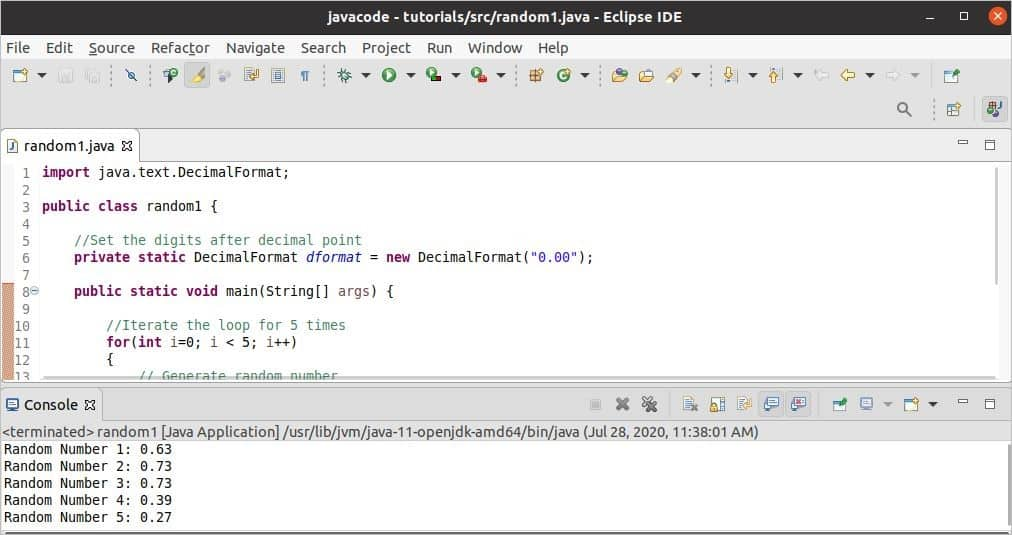### Example-2: Generate integer random number using Math.random

The following example shows how you can generate five random integer numbers by using Math.random class. Here, each random value is multiplied by 100 to get the number of 2 digits before the decimal point, and Math.round() method is used to get the integer value.

public class random2 {

public static void main(String[] args) {

System.out.println("Random number after conversion:");

//Iterate the loop for 5 times
for(int i=0; i < 5; i++)
{
//Generate random number and convert to long
long randnum = Math.round(Math.random()*100);

//Print the random value
System.out.println(randnum);
}
}
}

Output:

The following output will appear after running the script. Here, five integer numbers of two digits are generated.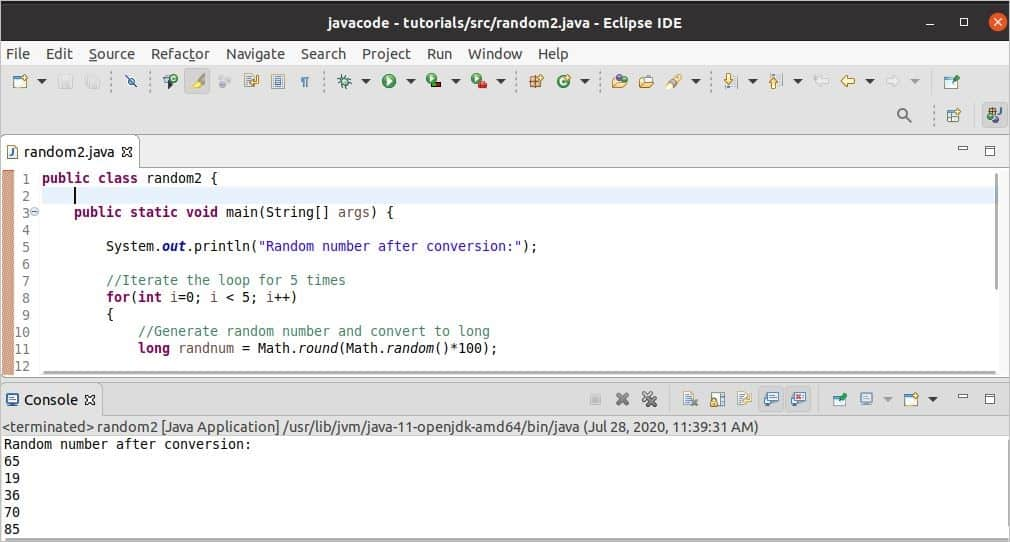### Example-3: Generate integer random number using Random class

You have to create the object of Random class to generate a random number using Random class that is shown in the following example. Here, the nextInt() method of Random class is used to generate 10 random integer numbers using the ‘for’ loop. According to the code, any number from 0 to 99 can be generated as a random number, but if any generated random number is more than 95, then the program will terminate from the loop.

import java.util.Random;
public class random3 {

public static void main(String[] args) {
//Declare the object
Random randObj = new Random();

//Iterate the loop 10 times
for (int i = 0; i  95)
{
System.out.println("The current number is more than 95");
break;
}

//Print the current random number
System.out.println("The current number is " + rNumber);
}
}
}

Output:

The output will vary each time you run code for the random number. The following output shows that a random number of more than 95 is generated after generating 5 random numbers and terminated from the loop.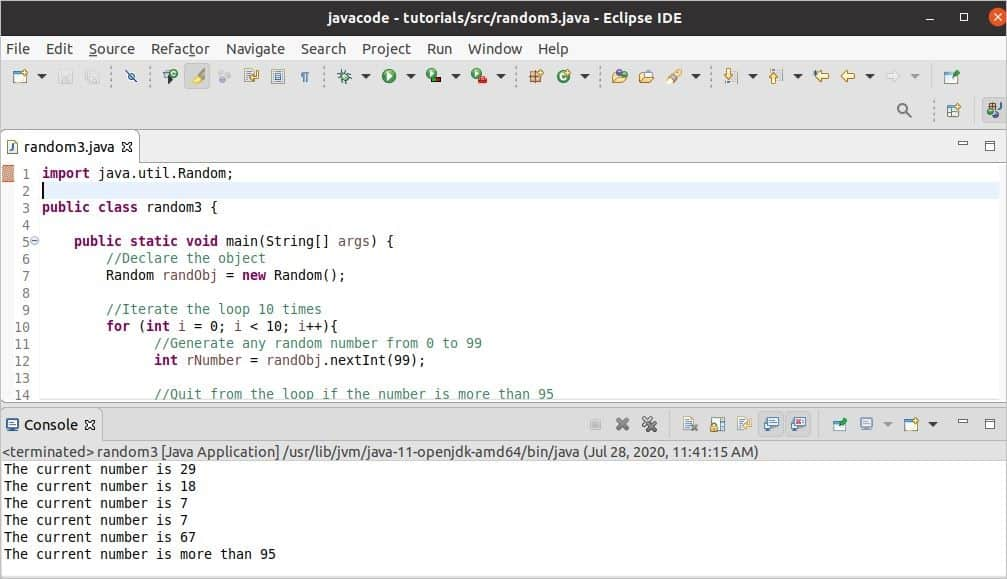### Example-4: Generate a random number within a range using Random class

The lower limit of generating random numbers using Random class is 0 by default. The following example shows how you can set the lower and upper limits before generating the random numbers. The lower limit and the upper limit will be taken as input from the user. Five random numbers will be generated within the range defined by the lower and upper limits.

import java.util.Random;
import java.util.Scanner;

public class random4 {

public static void main(String[] args) {

//Create a Scanner object
Scanner in = new Scanner(System.in);

//Set the lower limit
System.out.print("Enter lower limit : ");
int low = in.nextInt();

//Set the upper limit
System.out.print("Enter upper limit : ");
int high = in.nextInt();

//Declare the object
Random randObj = new Random();

//Iterate the loop 5 times
for (int i = 0; i < 5; i++){
//Generate any random number between low and high
int rNumber = randObj.nextInt((high - low) + 1) + low;

//Print the current random number
System.out.println("The current number is: " + rNumber);
}
//Close the scanner object
in.close();
}
}

Output:

10 is taken as a lower limit, and 50 is taken as an upper limit in the following output, and five random numbers are generated within this range.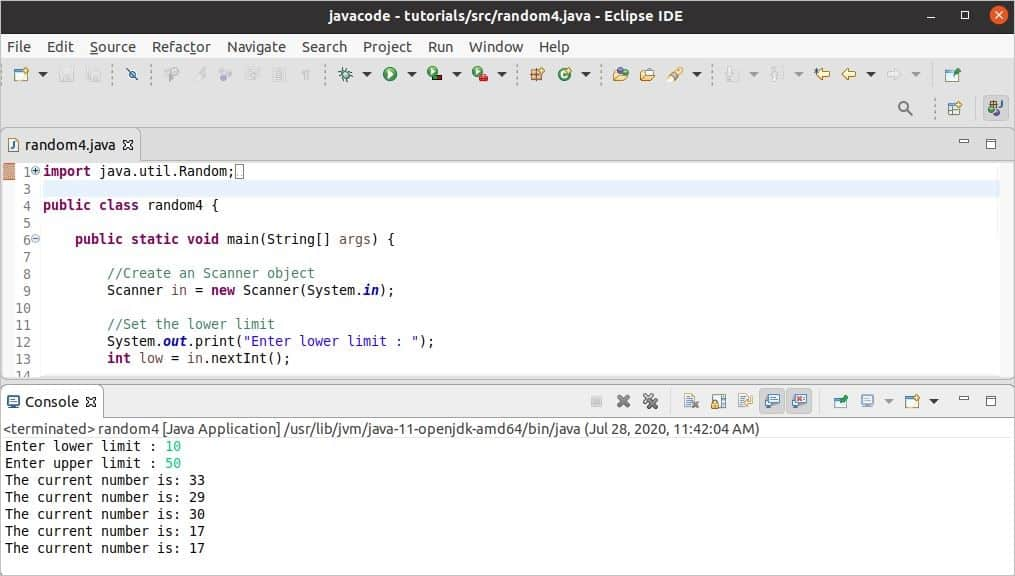### Example-5: Generate Boolean random values using Random class

Boolean random value can be generated by using the nextBoolean() method of Random class. The following example shows how three boolean values can be generated randomly by using the nextBoolean() method and ‘for’ loop.

import java.util.Random;

public class random5 {

public static void main(String[] args) {

//Declare the object
Random randObj = new Random();

System.out.println("Generated Random Boolean values are:");

//Iterate the loop for 3 times
for(int i=0; i < 3; i++)
{
//Generate any boolean value
Boolean rValue = randObj.nextBoolean();

//Print the current random value
System.out.println(rValue);
}
}
}

Output:

The output of the code can vary for generating value randomly. Three true values are generated randomly in the following output.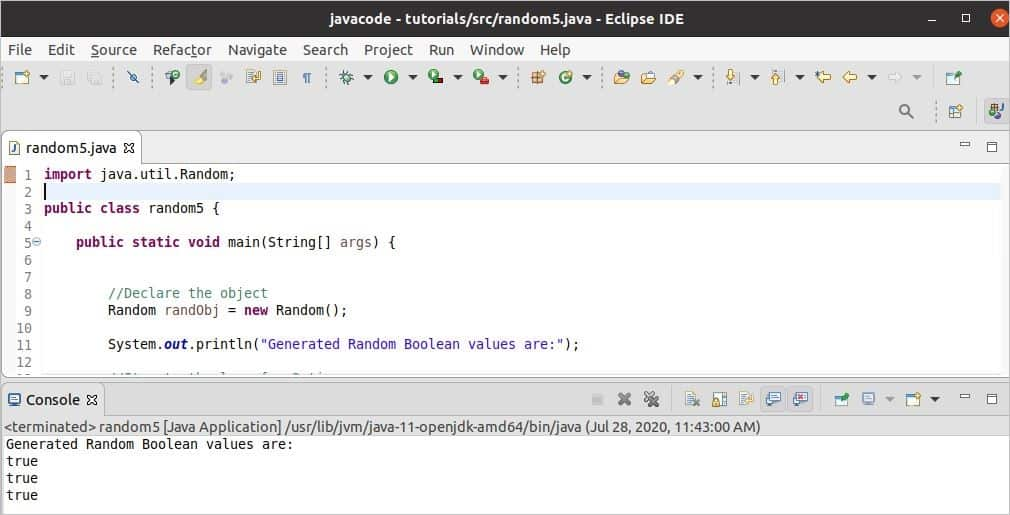### Conclusion:

Generating a random number is useful for different programming-related tasks, such as probability checking, lottery ticket generation, etc. Different ways to generate different types of random numbers are explained by using two Java classes in this tutorial. After practicing the examples of this tutorial, the concept of the random number will be cleared for the java users, and they will be able to generate random numbers as their programming requirement.